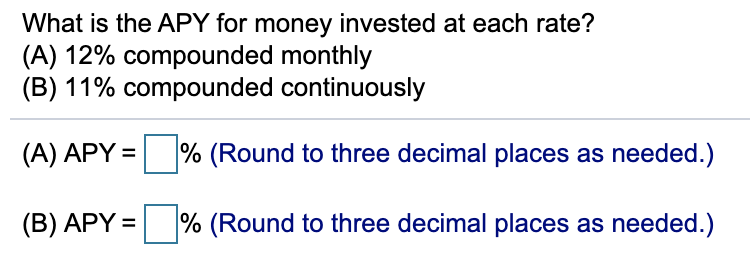# What is the APY for money invested at each rate?(A) 12% compounded monthly(B) 11% compounded continuously(A) APY =% (Round to three decimal places as needed.)% (Round to three decimal places as needed.)(B) APY =

Question
10 viewshelp_outlineImage TranscriptioncloseWhat is the APY for money invested at each rate? (A) 12% compounded monthly (B) 11% compounded continuously (A) APY = % (Round to three decimal places as needed.) % (Round to three decimal places as needed.) (B) APY = fullscreen
check_circle

Step 1

The formula for APY is

Step 2

a) Here, r=12%=0.12

n=12 ( compounded monthly).

Calculate APY.

Step 3

b) Here, r=11%=0.11

n=365 ( compounded con...

### Want to see the full answer?

See Solution

#### Want to see this answer and more?

Solutions are written by subject experts who are available 24/7. Questions are typically answered within 1 hour.*

See Solution
*Response times may vary by subject and question.
Tagged in

### Math